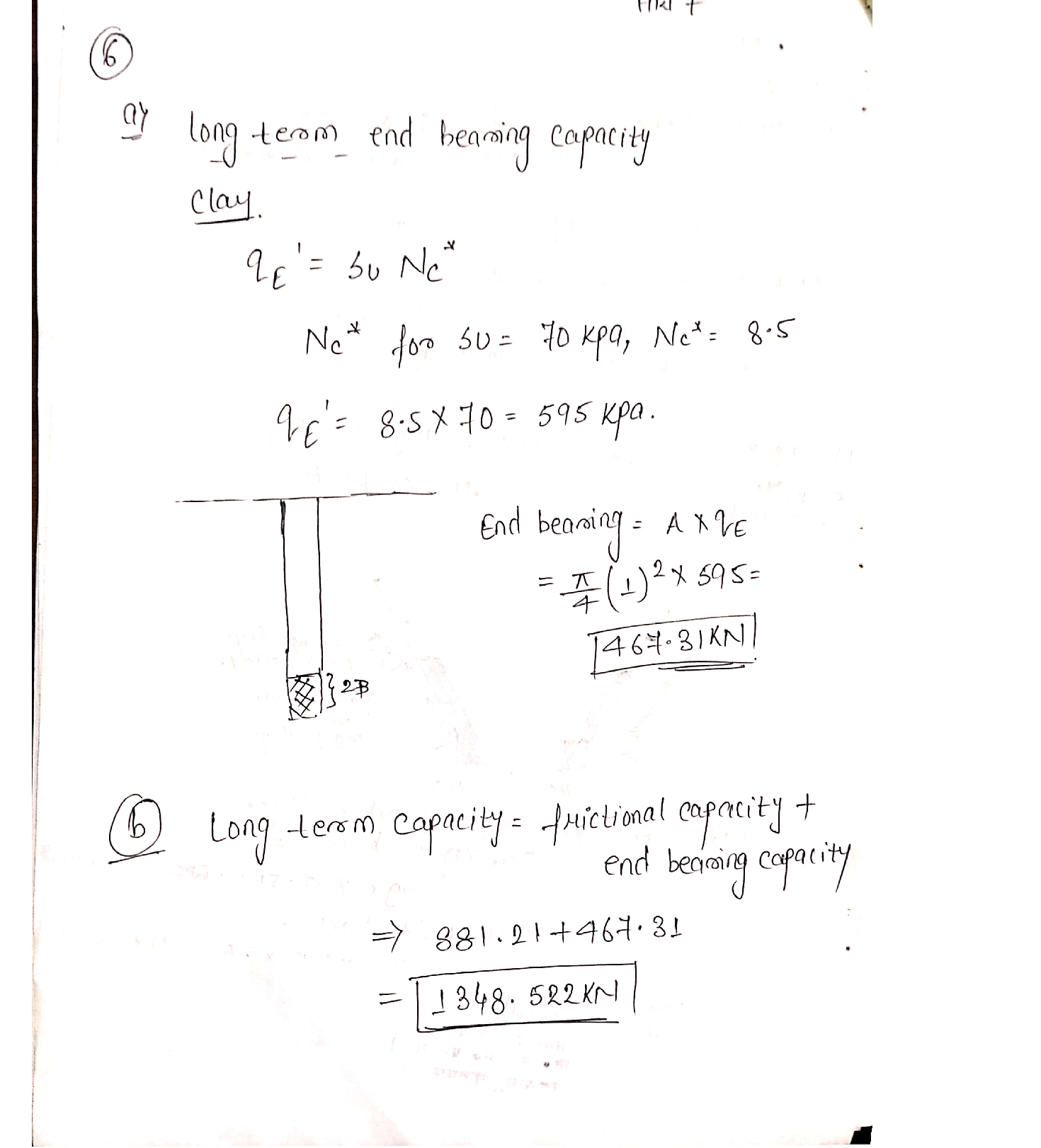Question

Geotechnical / Foundation Engineering

6. Study Deep Foundations video #11 and take notes on your Reader. Then,

а.Determine the long term end bearing capacity of the pile described in problem 4.

b. Determine the long term capacity of the pile described in problem 4.Verified### Question 45698Geotechnical / Foundation Engineering

9. What would the FS for the potential failure surface shown above be if the soil's undrained shear strength were 50 kPa (instead of 100 kPa)? Answer: FS = 0.98

### Question 45697Geotechnical / Foundation Engineering

8., Find the factor of safety for the failure surface shown, below using the Swedish Slip Circle method. The scale is in meters (use a ruler). The center of the circle is denoted by the •. Answer: FS 1.9550

### Question 43569Geotechnical / Foundation Engineering

50 tons as shown in Figure P8.11. Determine the averagestress increase beneath the center of the foundation in theA square foundation, 5 ft wide, carries a net column load ofAsquareclay layer:
a. Using Eq. (8.25),
b. Using Eqs. (8.26) and (8.10), and
c. Using Eqs. (8.26) and (8.15).

### Question 43568Geotechnical / Foundation Engineering

Three continuous foundations are shown in Figure P6.15.For each of them, what values would you use for their eccentricity and inclination in the bearing capacity calculations?

### Question 43567Geotechnical / Foundation Engineering

A 2 m x 3 m spread footing placed at a depth of2 m carries a vertical load of 3000 kN and a moment of300 kN m, as shown in Figure P6.14. Determine the factor of safety using Meyerhof's effective area method.

### Question 43566Geotechnical / Foundation Engineering

For an eccentrically loaded continuous foundation on sand,given B= 108 m Df=0.9 m e/B =0.12( one way eccentricity Y=16KN/m3 and theta =35 using the reduction factor method estimate load per unit length of the foundation

### Question 43565Geotechnical / Foundation Engineering

Repeat Problem 6.11 using Prakash and Saran's method.

### Question 43564Geotechnical / Foundation Engineering

An eccentrically loaded foundation is shown in Figure P6.11.Use FS of 4 and determine the maximum allowable load that the foundation çan carry, Use Meyerhof's effective area method.

### Question 43563Geotechnical / Foundation Engineering

For the design of a shallow foundation, given the following:
\phi^{\prime}=20^{\circ}
c^{\prime}=72 \mathrm{kN} / \mathrm{m}^{2}
\text { Unit weight, } \gamma=17 \mathrm{kN} / \mathrm{m}^{3}
Modulus of elasticity, E, = 1400 kN/m-%3D
\text { Poisson's ratio, } \mu_{s}=0.35
Foundation:L= 2 m
B = 1 m
D, = 1 m
Calculate the ultimate bearing capacity. Use Eq. (6.42).

### Question 43562Geotechnical / Foundation Engineering

A column foundation (Figure P6.9) is 3 m X 2 m in plan.Given: D; = 1.5 m, 6' = 25°, c' = 70 kN/m?. UsingEq. (6.28) and FS[see Eq. (6.24)] the foundation could carry.= 3, determine the net allowable load

### Submit query